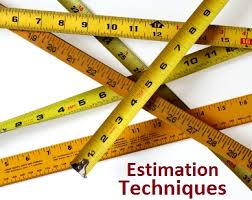7. Can you explain how TPA works?
There are three main elements which determine estimates for black box testing: size, test strategy, and productivity. Using all three elements we can determine the estimate for black box testing for a given project. Let’s take a look at these elements.
Size: The most important aspect of estimating is definitely the size of the project. The size of a project is mainly defined by the number of function points. But a function point fails or pays the least attention to the following factors:
Complexity: Complexity defines how many conditions exist in function points identified during a project. More conditions means more test cases which means more testing estimates. 208-1 (1)
Interfacing: How much does one function affect the other part of the system? If a function is modified then accordingly the other systems have to be tested as one function always impacts another.
Uniformity: How reusable is the application? It is important to consider how many similar structured functions exist in the system. It is important to consider the extent to which the system allows testing with slight modifications.

Test strategy: Every project has certain requirements. The importance of all these requirements also affects testing estimates. Any requirement importance is from two perspectives: one is the user importance and the other is the user usage. Depending on these two characteristics a requirement rating can be generated and a strategy can be chalked out accordingly, which also means that estimates vary accordingly.
Productivity: This is one more important aspect to be considered while estimating black box testing. Productivity depends on many aspects. 209-1

8. Can you explain steps in function points?

Below are the steps in function points:
First Count ILF, EIF, EI, EQ, RET, DET, FTR and use the rating tables. After you have counted all the elements you will get the unadjusted function points.
Put rating values 0 to 5 to all 14 GSC. Adding total of all 14 GSC to come out with total VAF. Formula for VAF = 0.65 + (sum of all GSC factor/100).
Finally, make the calculation of adjusted function point. Formula: Total function point = VAF * Unadjusted function point.
Make estimation how many function points you will do per day. This is also called as “Performance factor”.On basis of performance factor, you can calculate Man/Days.

9. Can you explain function points?
Function points are a unit measure for software much like an hour is to measuring time, miles are to measuring distance or Celsius is to measuring temperature. Function Points are an ordinal measure much like other measures such as kilometers, Fahrenheit, hours, so on and so forth.
This approach computes the total function points (FP) value for the project, by totaling the number of external user inputs, inquiries, outputs, and master files, and then applying the following weights: inputs (4), outputs (5), inquiries (4), and master files (10). Each FP contributor can be adjusted within a range of +/-35% for a specific project complexity.